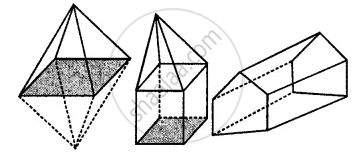# Verify Euler’S Formula for the Following Three-dimensional Figures: - Mathematics

Sum

Verify Euler’s formula for the following three-dimensional figures:#### Solution

(i)

Number of vertices = 6
Number of faces = 8
Number of edges = 12
Using Euler formula,
F + V – E = 2
8 + 6 – 12 = 2
2 = 2 Hence proved.

(ii)

Number of vertices = 9
Number of faces = 8
Number of edges = 15
Using, Euler’s formula,
F + V – E = 2
9 + 8 – 15 = 2
2 = 2 Hence proved.

(iii)

Number of vertices = 9
Number of faces = 5
Number of edges = 12
Using, Euler’s formula,
F + V – E = 2
9 + 5 – 12 = 2
2 = 2 Hence proved.

Concept: Euler's Formula
Is there an error in this question or solution?

#### APPEARS IN

Selina Concise Mathematics Class 8 ICSE
Chapter 19 Representing 3-D in 2-D
Exercise 19 | Q 4 | Page 220